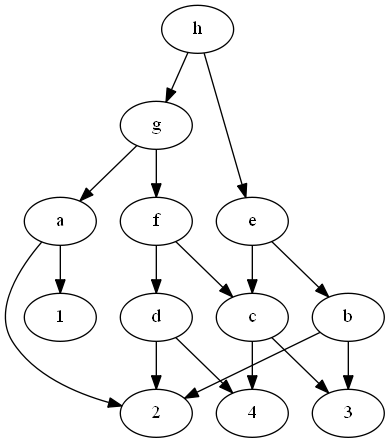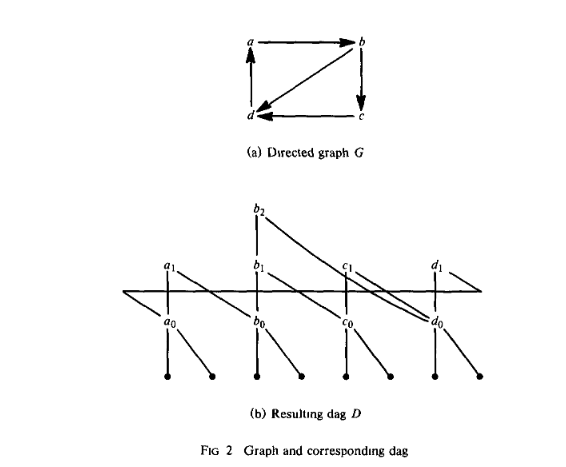# Tag Archives: Code Generation on a One-Register Machine

## Code Generation With Unlimited Registers

The reason I found the easier reduction for the previous problem is because it was in the paper that discussed this similar problem.

The problem: Code Generation With Unlimited Registers.  This is problem PO5 in the appendix

The description: Given a Directed, Acyclic graph G=(V, A), where no vertex has an out-degree larger than 2.    Just like the previous problem, the leaves of this graph (with in-degree 0) are our starting values, and the roots of the graph (with out-degree 0) are the final values we need to compute.

The operations that are allowed are:

• ri <- rj (copy a value between registers)
• ri <- ri op rj  (replace a value in ri with the combination of ri and rj.  This is how we “compute” nodes in the graph with 2 children)

(The problem definition in G&J makes a big deal about labeling the (at most) 2 edges leaving a vertex v as “Left” and “Right”, and so the ri comes from the “Left” side, and the rj comes from the “Right” side.  But G&J also say (as a “private communication”) that this problem remains NP-Complete if we allow commutative instructions- in other words, ri <- rj op ri is also allowed.  So I don’t think it matters.)

Can we compute the root vertices of G in K or fewer instructions, if we allow any number of values to be stored in registers?

Example: Here’s the complicated graph from last time:We can start by loading the 1,2,3, and 4 into registers 1-4.  Then we can do:

• R1 <- R1 op R2 (compute a)
• R2 <- R2 op R4 (compute d)
• R3 <- R3 op R4 (compute c)
• R2 <- R2 op R3 (compute f)
• R1 <- R1 op R2 (compute g)
• R5 <- 2 (another copy)
• R6 <- 3 (another copy)
• R5 <- R5 op R6 (computing b)
• R3 <- R3 op  R5 (computing e)
• R1 <- R1 op R3 (computing h)

The reason why this is hard, even with as many registers as we want, is because the operations are “accumulator” operations- they always put the result of the operation back into one of the operand registers, killing the old value.  So if we want to use that value more than once, we either need to reload it or be very careful about the order in which we compute vertices.

If we allowed 3-register operations like rk = ri op rj, then this is easily solvable by computing values “up the graph” in basically any bottom-up order you want, putting each vertex’s value into a new register.

Reduction: This is in the paper by Aho, Johnson, and Ullman that we used last time also talks about this variant of the problem.  They say that it’s basically the same situation, you just need to load the lead values into registers right away, and you get a situation that is “similar to” the one-register machine.  I think that the basic structure of the chains we are building still holds in this new situation.

Difficulty The one-register problem is a 6 or a 7, this is probably a 5 or 6 once you have that.  If you are going to make the students walk through the whole reduction step by step in this new situation, it might be a bit harder.

## Code Generation on a One-Register Machine

I’ve done a lot of traveling over the past month, so I’m sorry about missing posts.  Things should be back to normal now.

The problem: Code Generation on a One-Register Machine.  This is problem PO4 in the appendix.

The description: Given a directed acyclic graph G = (V,A), in which no vertex has an out-degree larger than 2, and a positive integer K.  The leaves of this graph (the vertices with out-degree 0) are our starting values, sitting in memory.  Can we compute the values in all root vertices  (with in-degree 0) in K instructions or less, if our only instructions are:

• Load a value from memory into our only register.
• Store a value from the register into memory
• Doing an operation combining a value in a register and a value in memory.  The operation must connect two children of a vertex in a graph together, and the “result” is the parent vertex.  The result value replaces the original value in the register.

Example:  Here’s a simple graph:Here, we can compute the “+” node by:

• Doing the + operation between the register and 2
• Storing the + operation to memory (G&J’s definition of the problem says that the node is not computed until the value is stored)

We can compute the “-” node in another 3 instructions, and since the value of the “-‘ node is still in the register, compute the “*” node in 1 more instruction, and store it with out last instruction.

Here’s a more complicated graph:To do this one, we will have to load the value in node 2 lots of times.  For example, here is the set of instructions I came up with to compute h:

• op to create a (only 1 operand)
• store a
• op to create c (3 is in memory)
• store c
• op to create d (4 is in memory)
• op to create f (c is in memory)
• op to create g (a is in memory)
• store g
• op to create b (3 is in memory)
•  op to create e (c is in memory)
• op to create h (g is in memory)
• store h

It’s possible that we can do this in fewer instructions, but hopefully, you can see why this problem is hard- knowing what value to keep in the register is tricky.

Reduction: G&J point out that the reduction is from a paper by Bruno and Sethi, which uses 3SAT to do the reduction.  The instance they build is pretty complicated.  I also came across a paper by Aho, Johnson, and Ullman, who extend the result of the Bruno and Sethi paper with a nice reduction from Feedback Vertex Set.  I think this reduction is easier to follow, so we’ll go with that.

So, we are given an instance of FVS- a directed acyclic graph G and an integer K.  We are looking for a set F of K vertices such that every cycle goes in G goes through some element of F.

We are going to build our Code Generation graph D as follows:

• For every vertex in G with outdegree d, build a “left chain” of d+1 vertices.  So if vertex a had 2 vertices leaving it, we will create 3 vertices b0, b1, and b2.  b2 will connect to b1, and b1 will connect to b0.
• Each of the “0” vertices at the bottom of these chains connects to 2 distinct memory values (they will be the leaves of the code graph)
• If vertex v has outdegree d, each vertex in a’s chain will connect to the different “0” vertex of the different neighbors of v in G.

Here is an example from the paper:Notice that if we don’t have the edges between the chains, we can compute the entire chain with just 2 loads (of the leaves that start in memory).  So, the only loads needed to compute all of D happen in the leaves, or in some of the “level 1” vertices that are parents of the leaves.   If we have to re-load one of those vertices, it is because there is no optimal strategy to avoid loading it, which means it’s part of a cycle.

For example, look at the a and b chains in the picture above.  If we didn’t have any of the c or d vertices or edges in our graph, we could compute a1 and b1 without loading any vertex that is not a leaf: compute b0, b1, b2, then a0, then a1 (which uses a0 from the register and b0 from memory).  The reason we can do this is that while a1 depends on b0, none of the b vertices depend on anything in a, which gives us a chain to do first.  We need to reload a value when we have a circular dependency between chains (so there is no correct chain to do first).  That’s the relationship between the chains and the feedback vertex set.

This works in the other direction as well- if we are given the feedback vertex set in G, we can compute those vertices first in D, and then load them all once as needed to compute D.

The paper says that in the example graph, the node {d} by itself is a Feedback Vertex Set, and the optimal computation ordering is: d0,c0, c1, b0,b1, b2, a0,a1, d1.  That final d1 needs a re-load of d0.  The 1 extra load corresponds to the 1 vertex in our Feedback Set.

Difficulty: 6.  Maybe 7.  I think this is right at the limit of what a student can figure out, but I would also want a more rigorous proof about the connection between the extra loads and the feedback set, which is probably tricky to come up with.